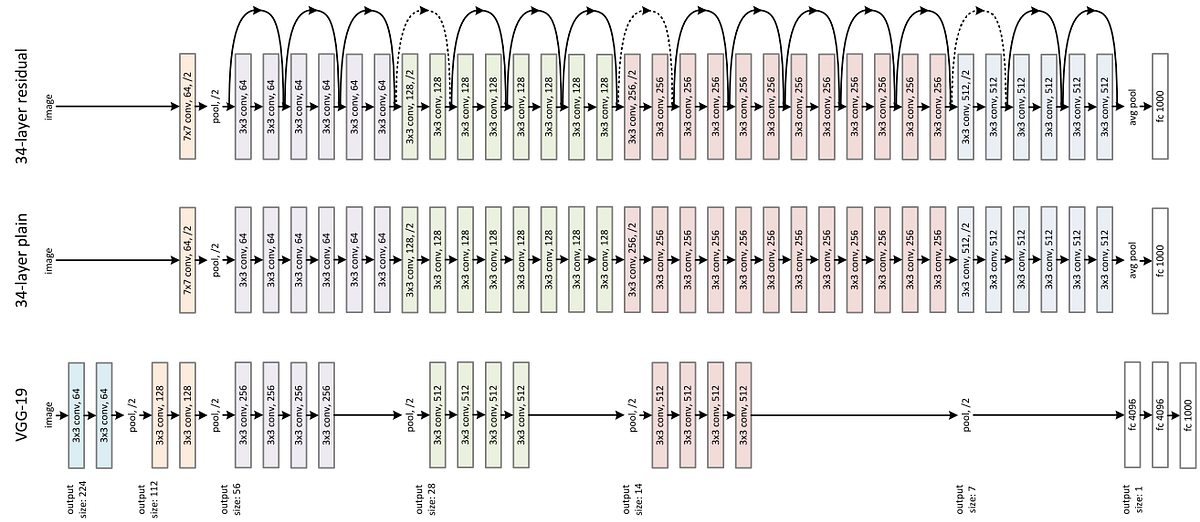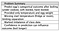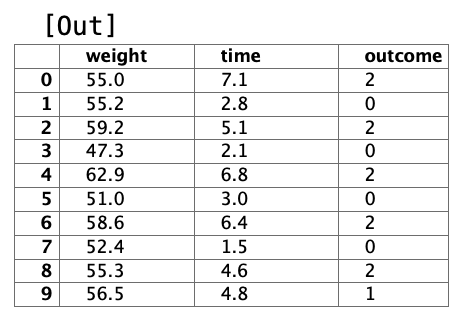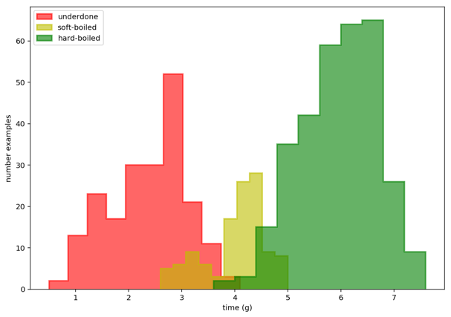1604157660

# A Deep Dive Into Residual Neural Networks

In this blog post, I’m going to present to you the **ResNet **architecture and summarize its paper, “Deep Residual Learning for Image Recognition” (PDF). I’ll explain where it comes from and the ideas behind this architecture, so let’s get into it!

## Introduction

At the time the ResNet paper got released (2015), people started trying to build deeper and deeper neural networks. This is because it improved the accuracy on the ImageNet competition, which is a visual object recognition competition made on a dataset with more than 14 million images.

But at a certain point, accuracies stopped getting better as the neural network got larger. That’s when ResNet came out. People knew that increasing the depth of a neural network could make it learn and generalize better, but it was also harder to train it. The problem wasn’t overfitting because the test error wasn’t going up when the training error was low. That’s why residual blocks were invented. Let’s see the idea behind it!

## The Idea Behind the ResNet Architecture

The idea behind the ResNet architecture is that we should at least be able to train a deeper neural network by copying the layers of a shallow neural network (e.g. a neural network with five layers) and adding layers into it that learn the identity function (i.e. layers that don’t change the output called identity mapping). The issue is that making the layer learn the identity function is difficult because most weights are initialized around zero, or they tend toward zero with techniques such as weight decay or l2 regularization.

#neural-networks #programming #deep-learning #machine-learning #artificial-intelligence

## Buddha Community1626106680

## Neural networks forward propagation deep dive 102

Forward propagation is an important part of neural networks. Its not as hard as it sounds ;-)

This is part 2 in my series on neural networks. You are welcome to start at part 1 or skip to part 5 if you just want the code.

So, to perform gradient descent or cost optimisation, we need to write a cost function which performs:

In figure 1, we can see our network diagram with much of the details removed. We will focus on one unit in level 2 and one unit in level 3. This understanding can then be copied to all units. (ps. one unit is one of the circles below)

Our goal in forward prop is to calculate A1, Z2, A2, Z3 & A3

Just so we can visualise the X features, see figure 2 and for some more info on the data, see part 1.

## Initial weights (thetas)

As it turns out, this is quite an important topic for gradient descent. If you have not dealt with gradient descent, then check this article first. We can see above that we need 2 sets of weights. (signified by ø). We often still calls these weights theta and they mean the same thing.

We need one set of thetas for level 2 and a 2nd set for level 3. Each theta is a matrix and is size(L) * size(L-1). Thus for above:

• Theta1 = 6x4 matrix

• Theta2 = 7x7 matrix

We have to now guess at which initial thetas should be our starting point. Here, epsilon comes to the rescue and below is the matlab code to easily generate some random small numbers for our initial weights.

``````function weights = initializeWeights(inSize, outSize)
epsilon = 0.12;
weights = rand(outSize, 1 + inSize) * 2 * epsilon - epsilon;
end
``````

After running above function with our sizes for each theta as mentioned above, we will get some good small random initial values as in figure 3

. For figure 1 above, the weights we mention would refer to rows 1 in below matrix’s.

Now, that we have our initial weights, we can go ahead and run gradient descent. However, this needs a cost function to help calculate the cost and gradients as it goes along. Before we can calculate the costs, we need to perform forward propagation to calculate our A1, Z2, A2, Z3 and A3 as per figure 1.

#machine-learning #machine-intelligence #neural-network-algorithm #neural-networks #networks1621440840

## Ultimate Guide for Deep Learning with Neural Network in 2021

In deep learning with Keras, you don’t have to code a lot, but there are a few steps on which you need to step over slowly so that in the near future, you can create your models. The flow of modelling is to load data, define the Keras model, compile the Keras model, fit the Keras model, evaluate it, tie everything together, and make the predictions out of it.

But at times, you might find it confusing because of not having a good hold on the fundamentals of deep learning. Before starting your new deep learning with Keras project, make sure to go through this ultimate guide which will help you in revising the fundamentals of deep learning with Keras.

In the field of Artificial Intelligence, deep learning has become a buzzword which always finds its way in various conversations. When it comes to imparting intelligence to the machines, it has been since many years that we used Machine Learning (ML).

But, considering the current period, due to its supremacy in predictions, deep learning with Keras has become more liked and famous as compared to the old and traditional ML techniques.

### Deep Learning

Machine learning has a subset in which the Artificial Neural Networks (ANN) is trained with a large amount of data. This subset is nothing but deep learning. Since a deep learning algorithm learns from experience, it performs the task repeatedly; every time it tweaks it a little intending to improve the outcome.

It is termed as ‘deep learning’ because the neural networks have many deep layers which enables learning. Deep learning can solve any problem in which thinking is required to figure out the problem.

### **Keras **

There are many APIs, frameworks, and libraries available to get started with deep learning. But here’s why deep learning with Keras is beneficial. Keras is a high-level neural network application programming interface (API) which runs on the top of TensorFlow – which is an end-to-end machine learning platform and is an open-source. Not just Tensorflow, but also CNTK, Theano, PlaidML, etc.

It helps in commoditizing artificial intelligence (AI) and deep learning. The coding in Keras is portable, it means that using Keras you can implement a neural network while using Theano as a backend and then subsequently run it on Tensorflow by specifying the backend. Also further, it is not mandatory rather, not needed at all to change the code.

If you are wondering why deep learning is an important term in Artificial Intelligence or if you are lagging motivation to start learning deep learning with Keras, this google trends snap shows how people’s interest in deep learning has been growing steadily worldwide for the last few years.

#deep learning #deep learning with neural network #neural network1598313600

## Introduction to Neural Networks

There has been hype about artificial intelligence, machine learning, and neural networks for quite a while now. I have been working on these things for over a year now so I would like to share some of my knowledge and give my point of view on Neural networks. This will not be a math-heavy introduction because I just want to build the idea here.

I will start from the neural network and then I will explain every component of a neural network. If you feel like something is not right or need any help with any of this, Feel free to contact me, I will be happy to help.

## When to use the Neural Network?

Let’s assume we want to solve a problem where you are given some set of images and you have to build an automated system that can categories each of those images to its correct label.

The problem looks simple but how do we come with some logic using raw pixel values and target labels. We can try comparing pixels and edges but we won’t be able to come with some idea which can do this task effectively or say the accuracy of 90% or more.

When we have this kind of problem where we have high dimensional data like Images and we don’t know the relationship between Input(Images) and the Output(Labels), In this kind of scenario we should use Neural Networks.ư

## What is the Neural network?

Artificial neural networks, usually simply called neural networks, are computing systems vaguely inspired by the biological neural networks that constitute animal brains. An ANN is based on a collection of connected units or nodes called artificial neurons, which loosely model the neurons in a biological brain

#artificial-intelligence #gradient-descent #artificial-neural-network #deep-learning #neural-networks #deep learning1602261660

## Deep Learning Explained in Layman's Terms

In this post, you will get to learn deep learning through a simple explanation (layman terms) and examples.

Deep learning is part or subset of machine learning and not something that is different than machine learning. Many of us, when starting to learn machine learning, try and look for the answers to the question, “What is the difference between machine learning and deep learning?” Well, both machine learning and deep learning are about learning from past experience (data) and make predictions on future data.

Deep learning can be termed as an approach to machine learning where learning from past data happens based on artificial neural networks (a mathematical model mimicking the human brain). Here is the diagram representing the similarity and dissimilarity between machine learning and deep learning at a very high level.

#machine learning #artificial intelligence #deep learning #neural networks #deep neural networks #deep learning basics1596720780

## Bayesian Neural Networks: 2 Fully Connected in TensorFlow and Pytorch

This chapter continues the series on Bayesian deep learning. In the chapter we’ll explore alternative solutions to conventional dense neural networks. These alternatives will invoke probability distributions over each weight in the neural network resulting in a single model that effectively contains an infinite ensemble of neural networks trained on the same data. We’ll use this knowledge to solve an important problem of our age: how long to boil an egg.

## Chapter Objectives:

• Become familiar with variational inference with dense Bayesian models
• Learn how to convert a normal fully connected (dense) neural network to a Bayesian neural network
• Appreciate the advantages and shortcomings of the current implementation

The data is from an experiment in egg boiling. The boil durations are provided along with the egg’s weight in grams and the finding on cutting it open. Findings are categorised into one of three classes: under cooked, soft-boiled and hard-boiled. We want the egg’s outcome from its weight and boiling time. The problem is insanely simple, so much so that the data is near being linearly separable¹⁠. But not quite, as the egg’s pre-boil life (fridge temperature or cupboard storage at room temperature) aren’t provided and as you’ll see this swings cooking times. Without the missing data we can’t be certain what we’ll find when opening an egg up. Knowing how certain we are we can influence the outcome here as we can with most problems. In this case if relatively confident an egg’s undercooked we’ll cook it more before cracking it open.Let’s have a look at the data first to see what we’re dealing with. If you want to feel the difference for yourself you can get the data at github.com/DoctorLoop/BayesianDeepLearning/blob/master/egg_times.csv. You’ll need Pandas and Matplotlib for exploring the data. (pip install — upgrade pandas matplotlib) Download the dataset to the same directory you’re working from. From a Jupyter notebook type pwd on its own in a cell to find out where that directory is if unsure.Figure 2.01 Scatter plot of egg outcomes

And let’s see it now as a histogram.Figure 2.02 Histogram of egg times by outcome

It seems I wasn’t so good at getting my eggs soft-boiled as I like them so we see a fairly large class imbalance with twice as many underdone instances and three times as many hardboiled instances relative to the soft-boiled lovelies. This class imbalance can spell trouble for conventional neural networks causing them to underperform and an imbalanced class size is a common finding.

Note that we’re not setting density to True (False is the default so doesn’t need to be specified) as we’re interested in comparing actual numbers. While if we were comparing probabilities sampled from one of the three random variables, we’d want to set density=True to normalise the histogram summing the data to 1.0.

#editors-pick #bayesian-machine-learning #deep-learning #bayesian-neural-network #neural-networks #deep learning